# Torque and power

Subjects:

• Motor torque
• Engine power
• Measuring torque and power
• Horsepower and kiloWatt

Motor Torque:
Engine torque is the force with which the engine crankshaft rotates. The torque arises from the combination of the combustion force on the piston and the distance from the crank radius. The force on the piston depends on, among other things, the filling degree (air quantity) and the fuel quantity, and varies because the angle of the force transmission to the crank pin is constantly changing. We can calculate the mean piston pressure from the indicator diagram or get a PV diagram.

In the following line drawing we see the piston being pushed down by the combustion force (p). This combustion pressure creates force F, the piston force. The piston force is transmitted to the crankshaft journal (r) via the connecting rod (S). This creates the so-called tangential force (Ft).

The torque is calculated using the formula Ft xr (the tangential force multiplied by the crank radius) and is expressed in Nm (Newton meters).Description:
p = pressure on the piston.
F = the force on the piston
N = Guideway Force
S = Force on the connecting rod
Ft = Tangential force

Due to the varying combustion pressure and the twisting of the crank-connecting rod mechanism, the tangential force is also not a constant quantity. We therefore work with an average tangential force.

We can determine the tangential force in when we decompose the piston force (see the image below and the page “dissolve piston force").

The engine torque depends solely on the force on the piston, because all other quantities such as the piston diameter and the crank radius are fixed engine data. The force on the piston (Fz) is negated by the combustion pressure (p) and depends on the filling degree of the engine (at stoichiometric mixing ratio). It is mainly the throttling in the intake manifold that determines the degree of filling of the engine.

The greatest throttling is caused by the position of the throttle valve. The throttle position has the greatest influence on the engine torque, because we influence the engine performance by changing the throttle position. In a test set-up, we measured the maximum torque delivered with the throttle fully open.

The torque is not the same everywhere at different speeds and fully open throttle. Due to the changing gas velocities and fixed valve opening angles, the torque will only be optimal at a certain speed.

In the images below we see the power and torque diagrams of two types of diesel engines used in a BMW 3 Series (E9x). With both engines, the torque is reached at about 1800 rpm, but is clearly higher with the 320d than with the 316d. Both engines have a displacement of 2.0 liters. The higher torque is made possible by, among other things, supercharging, valves in the intake manifold and the mapping of the engine management system, which determines consumption and exhaust emissions in addition to torque.

Engine power:
In the factory specifications, in addition to the engine torque, the engine power is also mentioned. Engine power is a multiplication of engine torque by engine speed. Power is actually how many times the torque can be delivered per second. The official formula is: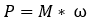where P is the power in Nm/s or Watt, M ​​is the torque in Nm and ω (omega) is the angular velocity. The letter T is also used for the couple instead of M.
Since the angular velocity (ω) is equal to 2 * π * n, where n is equal to the number of revolutions per second, we can change the formula to: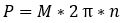As an example we take a naturally aspirated four-cylinder 2.0 liter FSI engine with four valves per cylinder from the VAG (engine code: AXW). Of course we can read the torque and power from the graph, but in this section we calculate the power from the torque.

Data:

• engine torque: 200 Nm;
• speed: 3500 rpm = 58,33 rpm

Wanted: the power delivered at the given speed.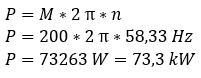The delivered torque and power at 3500 rpm are 200 Nm and 73,3 kW.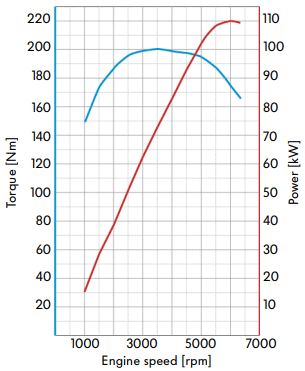Measuring torque and power:
The torque is directly responsible for the pulling power of the car. The torque is multiplied by the transmission ratio (i) of the gearbox and final reduction, and divided by the loaded radius (rb) of the driving wheels (see page calculate gear ratios).

Engine torque is measured by braking the engine at various speeds with the throttle fully open. By braking the engine, the selected speed is kept constant. The braking force of the motor, multiplied by the radius of the measuring object on which the force acts, is then the motor torque.

An eddy current brake can be used for power measurement. The measurement takes place directly on the crankshaft. Here, electromagnets generate eddy currents in a metal disc, where the braking force is determined by measuring the bending of a torsion element. In a power measurement of a motor on an eddy current brake, the speed and torque are the measured quantities. The power is determined by means of a calculation (see the previous paragraph).

The power of a vehicle can also be measured directly at the wheels. Losses of up to 70% must be taken into account here. These losses arise in the transmission. The axle power (the power measured on the wheels on the power test bench) is also called the DIN horsepower. The power measured at the flywheel is called the SAE horsepower. SAE stands for Society of Automotive Engineers. The value of the SAE will therefore always be higher than that of the DIN.

The metal rollers of the test bench are connected to a braking mechanism, usually with an eddy current brake. The force with which the rollers are decelerated, together with the speed of both the wheels and the crankshaft, measures the torque delivered and the power is calculated. The measurement is usually made in the highest or second highest gear with the accelerator pedal fully depressed. A loss of 15 to 30% is not uncommon for two-wheel drive vehicles. The dynamometer computer compensates for this loss by measuring how much power it takes to run the dynamometer to drive the vehicle. In this measurement, the vehicle rolls out with the clutch depressed.

Engineers or tuners try to keep the torque curve as flat as possible, so that the engine torque remains the same over as many rpms as possible. Especially engines with supercharging (turbo / compressor) that significantly increase the torque, can be adjusted as flat as possible in this way. Also by applying filling degree-increasing techniques, such as multi-valve engines, variable valve timing or a variable intake manifold the coupling area can be kept as flat as possible.

If we were to measure the torque at different throttle positions, we would get a gradient like the following figure. However, such a measurement is rarely performed.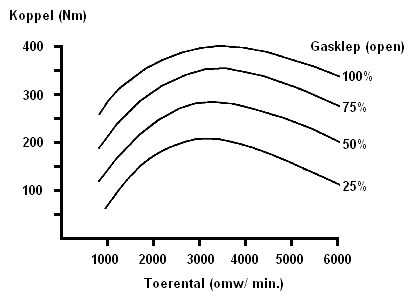Horsepower (hp) and kiloWatts (kW):
To express the power of a vehicle, the units “horsepower” and “kilowatt” are used. The power depends on the torque per second. The definition of horsepower comes from the time when transport was still by horse and carriage. If a mass of 75 kilograms is lifted over a distance of 1 meter within 1 second, a power of 1 horsepower is delivered. So 1 hp is 75 kg * 1 meter / 1 second.

If we look at the power from the unit Watt, then 1 Watt is a multiplication of 1 Newton * 1 meter per second. We abbreviate this as [1 Nm / sec].

The horsepower (hp) used in the Netherlands is exactly the same as the German Pferdestärkte (PS) and the French Chaval-Vapeur (CH).
1 hp = 0,7355 kW
1 kW = 1,3596 hp

The English/American horse power (hp) is greater.
1 hp = 0,7457 kW
1 kW = 1,3410 hp

If we convert horsepower to Watt, we have to multiply the mass by the acceleration of gravity: 1 HP = 75 kg/sec * 9,81 m/s^2 = 7355 W = 0,7355 kW.
To convert the power of a 150 hp engine, we multiply the number of kg/sec. with horsepower. This results in: (150 * 75) * 9,81 = 110,4 kW.

We can also convert the power in Watts to horsepower. We do this as follows: 1 / 0,7355 (W) = 1,36 hp. A motor with a power of 92 kW delivers according to the calculation: (1 * 92) / 0,736 = 125 hp.error: Alert: Content is protected !!